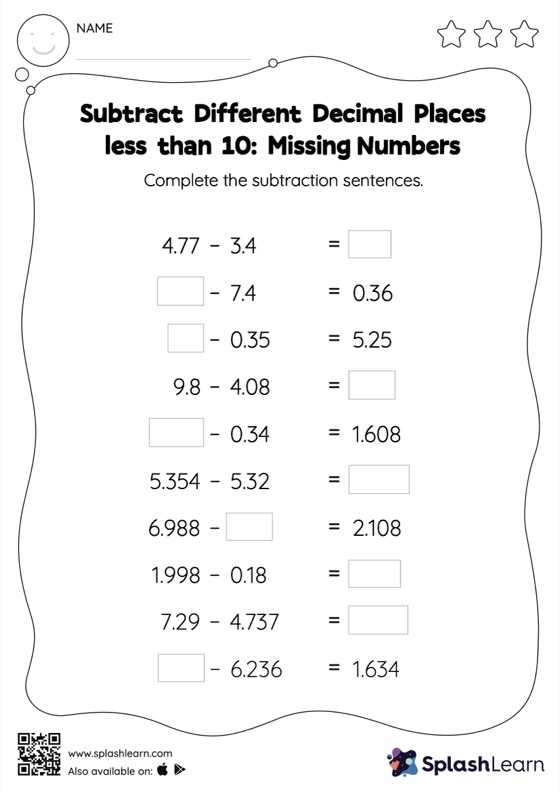# Subtract Different Decimal Places less than 10: Missing Numbers Worksheet

Home > Subtract Different Decimal Places less than 10: Missing NumbersThis worksheet gives students an opportunity to apply the concepts of subtraction to subtract different decimal places less than 10. As the worksheet uses a horizontal format, it allows for more creativity when it comes to how the student solves the problem. In contrast, in the vertical format, students usually employ the standard method to solve.